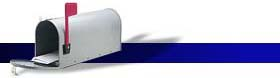####Syllabus: Introduction to Statistics and Probability (Math 111)

Learning Objectives:

• To acquaint the student with the fundamental applications of statistics and probability
• To learn about experiments, outcomes, probabilities, and odds
• To learn about probability distributions, including - binomial, normal, and sampling distributions
• To provide a foundation for the study of more specialized methods

Textbook: Larson, Ron; and Farber, Betsy; Elementary Statistics (Fourth Edition); Pearson, Prentice Hall; ISBN 13:978-0-13-242433-2 and ISBN 10: 0-13-242433-9

• All students are expected to participate in the learning experience.
• All exams are equally weightedCourse Outline

Chapter 1

Introduction to Statistics, sections 1-3.
Course overview, Overview of statistics, Data classification, Experimental design, Chapter summary and review.

Chapter 2

Descriptive Statistics, sections 1-5.
Frequency distributions and their graphs, More graphs and displays, Measures of central tendency, Measures of variation, Measures of position, Chapter summary and review.

Chapter 3

Probability, sections 1-3.
Basic concepts of probability and counting, Conditional probability and the multiplication rule, The addition rule, Additional topics in probability and counting. Chapter summary and review.

Chapter 4

Discrete Probability Distributions, sections 1-3.
Probability distributions, Binomial distributions, More discrete probability distributions, Chapter summary and review.

Chapter 5

Normal Probability Distributions, sections 1-5.
Introduction to normal distributions and the standard normal distribution, Finding probabilities, Finding values, Sampling distributions and the central limit theorem, Normal approximations to binomial distributions, Chapter summary and review.

Chapter 6

Confidence Intervals, sections 1-4.
Confidence intervals for the mean (large samples and small samples), for population proportions and for variance and standard deviation. Chapter summary and review.

Chapter 7

Hypothesis Testing with One Sample, sections 1-5.
Introduction to hypothesis testing, Hypothesis testing for the mean (large and small samples), for proportions and for variance and standard deviation. Chapter summary and review.

Chapter 8

Hypothesis Testing with Two Samples, sections 1-4.
Testing the difference between means (both large independent samples and small independent samples), Testing the difference bwween means (dependent samples), Testing the difference between proportions. Chapter summary and review.

Chapter 9

Correlation and Regression, sections 1-4.
Correlation, Linear regression, Measures of regression and prediction intervals, Multiple regression. Chapter summary and review.

Chapter 10

Chi-Test and the F-Distribution, sections 1-4.
Goodness of fit, Independence, Comparing two variances, Analysis of variance. Chapter summary and review.

Chapter 11

This chapter will be covered as time allows.